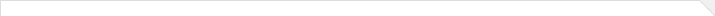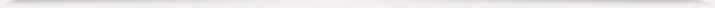报告题目： Random perturbation of low-rank matrices 报 告 人： 王可 助理教授 报告人所在单位： 香港科技大学 报告日期： 2021-08-25 星期三 报告时间： 14:30--15:30 报告地点： 腾讯会议 ID: 470 256 947 报告摘要： Computing the singular values and singular vectors of a large matrix is a basic task in high dimensional data analysis with many applications in computer science and statistics. In practice, however, data is often perturbed by noise. In this talk, we consider the matrix model Y=S+X where S is a low-rank deterministic matrix, representing the signal, and X is random noise. We investigate how much the singular value or singular vector of S is altered by a random perturbation. We show that better estimates can be achieved under this setting, improving classical perturbation bounds. We also give a precise description of the limiting distribution of the angles between the outlier singular vectors of Y with their counterparts, the leading singular vectors of S. It turns out that the limiting distribution depends on the structure of S and the distribution of X, and thus it is non-universal. 本年度学院报告总序号： 216